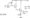# Analog Electronic Circuits December 2010

Note: Answer any FIVE full questions, selecting at least TWO Questions from each of Part -A and Part-B.

PART-A

1  a. What is an equivalent circuit of a device? Explain the different equivalent circuits for semiconductor diode.

b.  Analyse the circuit shown below, Fig. Ql(b), and draw the output waveform. Assume Vr – 0,7V.

c. Write a suitable circuit to get the following transfer characteristics fig. Ql (c), and explain its working.

2 a. Find Ic and VCE for the circuit shown, Fig. Q2(a).b.  Find the coordinates of the Q point and locate it on the dc load line for the voltage divider configuration. Given Vcc = 16V, Ri (upper resistor) = 62 kQ., R2 = 9.1 k£l, Rc = 3.9kQ , Re – 0.68kQ and P = 80. The coupling capacitors are 10µF each. Also find Vc, VE and VB.

c.   Define turn ON time and turn OFF time of a transistor. Design a transistor inverter if Vcc = 10V, Ic = 10mA and p = 250. Assume input to be a pulse of amplitude 10V.

3 a. What is bias stabilization? Explain. Derive an expression for S(Ico) and S(VBE) for fixed bias configuration.

b.  For an emitter bias circuit (capacitor is bypassed), determine re, Zj, Z0 and Av. Given Rb = 470 kH , Rc = 2.2kQ, Vcc = 20V, RE = 0.56kQ , CE = 10µF , p = 120 , r0 = 40kQ , Cc = lO^F.

c.   Determine i) the common logarithm of the number 2.2x 103. ii) the power gain in decibels for P0 = 100 m watts ,Pi = 5m watts.

4 a.The transistor is connected as a CE amplifier. Determine Zc, Z0, Ai and Ay using complete hybrid model.

b.  Discuss the low frequency and high frequency response of a RC coupled amplifier.

PART-B

5  a. For the circuit of fig. Q5(a), calculate the dc bias voltage Ve-b.  With a block diagram, explain the difference between voltage series and voltage shunt feedback.

c.   Using the block diagram approach, derive an expression for Af and Z0f for current series feedback amplifier.

6 a. With a neat circuit diagram, explain the operation of a transformer coupled class A power amplifier.

b.  For a class B amplifier with Vcc ~ 25V driving an 80 load, determine i) maximum I/P power ii) maximum O/P power iii) maximum circuit efficiency.

c.   Calculate the second harmonic distortion for an O/P waveform having measured values of

7 a. With a neat circuit diagram, explain the working principle of RC phase shift oscillator, with relevant equations.

b.  What are the tuned oscillators? Explain any one type of tuned oscillator.

8 a. Define gm and rd of field effect transistor. Explain the procedure to determine the above values graphically.

b. Write the ac equivalent circuit for voltage divider JFET configuration and determine Zj, Z0 and Av.

c.   Differentiate between enhancement and depletion MOSFET.

### 1 thought on “VTU Previous Year Question Papers BE EC Third Semester Analog Electronic Circuits December 2010”

1.3rd semester vtu previous year question papers of Analog Electronic circuits 2011,2012